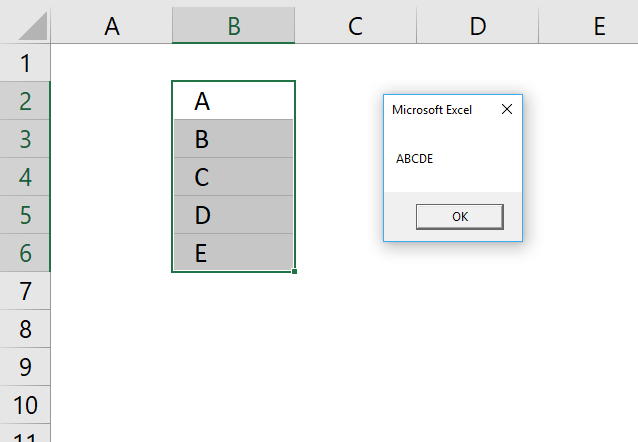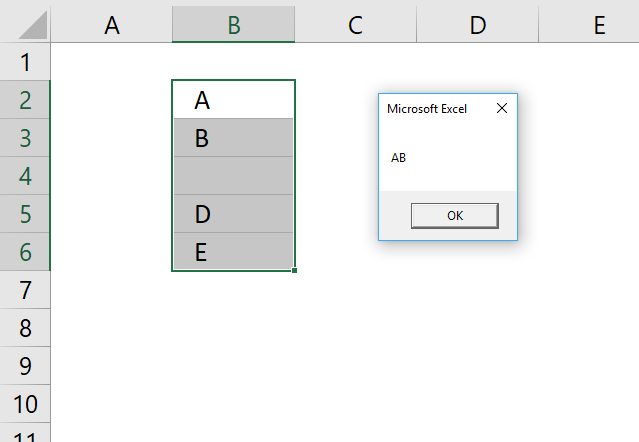Author: Oscar Cronquist Article last updated on February 01, 2018The Do Loop statement allows you to repeat specific code until a condition is met.

There are two possible ways you can use Do Loop with the While statement, here is the first one.

#### Statement Syntax

DO WHILE [condition]
[CODE]
LOOP

As you can see the While statement is after the Do statement. This makes it possible to avoid the Do Loop altogether if the condition is not met.

#### Example macro

The macro below, demonstrated in above picture, concatenates values from cell range B2:B6 as long as variable r is smaller than 7.

```Sub Macro1()
r = 1
Do While r < 6
result = result & Range("B2:B6").Cells(r, 1)
r = r + 1
Loop
MsgBox result
End Sub
```

You can all use the While statement after the Loop statement, this makes the macro go through the Do Loop statement at least one time.

#### Statement Syntax

DO
[CODE]
LOOP WHILE [condition]

#### Example macro

```Sub Macro2()
r = 1
Do
result = result & Range("B2:B6").Cells(r, 1)
r = r + 1
Loop While r < 6
MsgBox result
End Sub
```

### Exit DoThe Exit Do allows you to stop a Do Loop before the condition is met.

The macro then continues with the line right below Loop, if there is one.

#### Statement Syntax

DO WHILE [condition]
IF [condition] THEN EXIT DO
[CODE]
LOOP

The following macro stops the Do Loop if cell value is empty, it then continues with the line just under Loop which is Messagebox.

This is why the message box displays only two concatenated values on the picture above, the third cell is empty.

#### Example macro

```Sub Macro3()
r = 1
Do While r < 6
If Range("B2:B6").Cells(r, 1) = "" Then Exit Do
result = result & Range("B2:B6").Cells(r, 1)
r = r + 1
Loop
MsgBox result
End Sub
```

### Do Until Loop

The While statement allows you to iterate through the Do Loop while the condition is true.

The Until statement runs the code until the condition is met.

#### Statement Syntax 1

DO UNTIL [condition]
[CODE]
LOOP

If the Until condition is not met the entire do loop structure is ignored, the macro continues with the row below Loop.

#### Example macro 1

```Sub Macro4()
r = 1
Do Until r = 6
result = result & Range("B2:B6").Cells(r, 1)
r = r + 1
Loop
MsgBox result
End Sub
```

#### Statement Syntax 2

DO
[CODE]
LOOP UNTIL [condition]

In this case the code between Do and Loop is rund at least once.

#### Example macro 2

```Sub Macro5()
r = 1
Do
result = result & Range("B2:B6").Cells(r, 1)
r = r + 1
Loop Until r = 6
MsgBox result
End Sub
```

### Get Excel *.xlsm file

How to use the Do Loop statement.xlsm